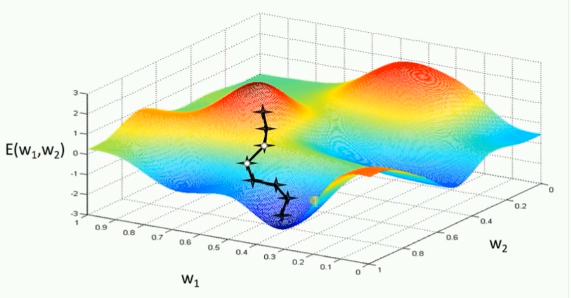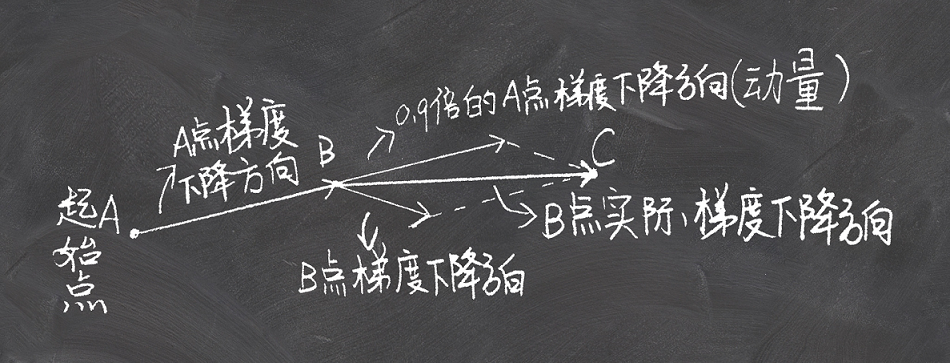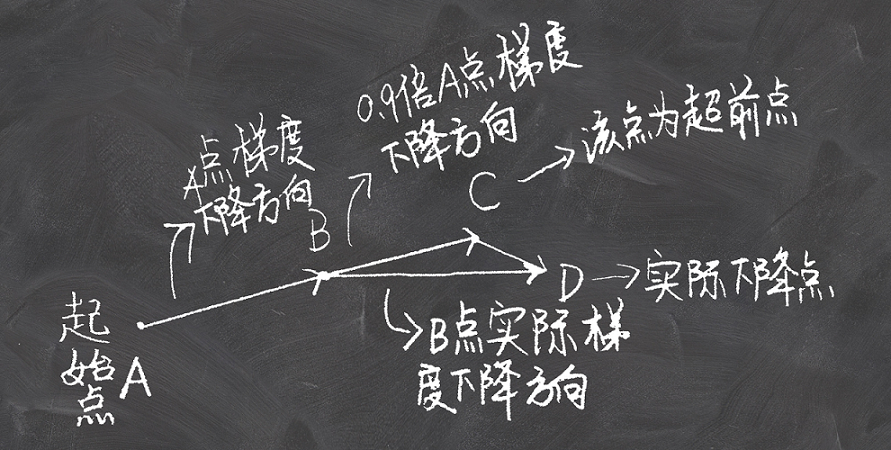## 0x00 标准梯度下降法（GD）

$W$
，代价函数为
$J(W)$
，则代价函数关于模型参数的偏导数即为相关梯度
$\Delta J(W)$
，学习率为
$\eta_t$
$W_t$

$t$

$W_{t+1}$

$W_{t+1}=W_t-\eta_t\Delta J(W_t)$1. 1.
训练速度慢：每走一步都要要计算调整下一步的方向，下山的速度变慢。在应用于大型数据集中，每输入一个样本都要更新一次参数，且每次迭代都要遍历所有的样本。会使得训练过程及其缓慢，需要花费很长时间才能得到收敛解。
2. 2.
容易陷入局部最优解：由于是在有限的视距内寻找下山的方向。当落入鞍点（局部最优解），梯度为0，使得模型参数不再继续更新。

## 0x01 批量梯度下降法（BGD）

$W_{t+1}=W_t-\eta_t\sum_{i=1}^n\Delta J(W_t, X_{(i)}, Y_{(i)})$

$X{(i)}, Y{(i)}$

## 0x02 随机梯度下降法（SGD）

$X{(i)}, Y{(i)}$

$W_{t+1}=W_t-\eta_t\Delta J(W_t, X_{(i)}, Y_{(i)})$

SGD的优点就是计算速度快，而且只要不是噪声特别大，SGD均能很好地收敛。缺点是随机选择梯度的过程会引入噪声，使得权值更新的方向不一定正确，此外SGD也没有客服局部最优解的问题。

## 0x03 $\Delta J(W)$ 详解

$\Delta J(W)$
，其意为代价函数
$J(W)$

$J=\frac{1}{2n}\sum_x(y-\text{prediction})^2 \\ \text{prediction}=\sigma(z) \\ z=WX+b$

$\frac{\partial J}{\partial W}=(y-\text{prediction})\sigma'(z)X \\ \text{prediction}=\sigma(z) \\ z=WX+b$

# 0x01 动量优化法（Momentum）

## 0x00 使用动量的随机梯度下降法

$v_t=\alpha v_{t-1}+\eta_t\Delta J(W_t, X_{(i)}, Y_{(i)}) \\ W_{t+1}=W_t-v_t$

$\alpha v_{t-1}$
，此处的
$\alpha$

$v_t$## 0x01 Nesterov Accelerated Gradient (NAG)$v_t=\alpha v_{t-1}+\eta_t\Delta J(W_t-\alpha v_{t-1}) \\ W_{t+1}=W_t-v_t$
$W_t-\alpha v_{t-1}$

$\alpha=0.9$
）A点的梯度，到达超前点C，然后在C点按照超前梯度进行合并。
NAG在Tensorflow中与Momentum合并在同一函数tf.train.MomentumOptimizer中，可以通过参数配置启用。

# 0x02 自适应学习率优化算法

$W_{t+1}=W_t-\frac{\eta}{\sqrt{G_t+\epsilon}}\Delta J(W_t) \\ G_t=G_{t-1}+\Delta_tJ(W_t)=\sum_{t'=1}^{t}\Delta_{t'}J(W_{t'})$

$\epsilon$

$G_t$

$G_t$

## 0x01 RMSProp算法

$W_{t+1}=W_t-\frac{\eta}{\sqrt{E[g^2]_t+\epsilon}}g_t \\ E[g^2]_t=\alpha E[g^2]_{t-1}+(1-\alpha)g_t^2 \\ g_t=\Delta J(W_t)$
$E[g^2]_t$

$E[g^2]_t=\alpha E[g^2]_{t-1}+(1-\alpha)g_t^2 \\ \Delta W_t=-\frac{\sqrt{\sum_{i=1}^{t-1}\Delta W_i}}{\sqrt{E[g^2]_t+\epsilon}} \\ W_{t+1}=W_t+\Delta W_t$

$\sum_{i=1}^{t-1}\Delta W_i$

$m_t=\beta_1m_{t-1}+(1-\beta_1)g_t,~ \hat{m}_t=\frac{m_t}{1-\beta_1^t} \\ v_t=\beta_2v_{t-1}+(1-\beta_2)g_t^2,~ \hat{v}_t=\frac{v_t}{1-\beta_2^t} \\ W_{t+1}=W_t-\frac{\eta}{\sqrt{\hat{v}_t}+\epsilon}\hat{m}_t$

$m_t$
$v_t$

$\beta_1$
$\beta_2$

$\hat{m}_t$
$\hat{v}_t$